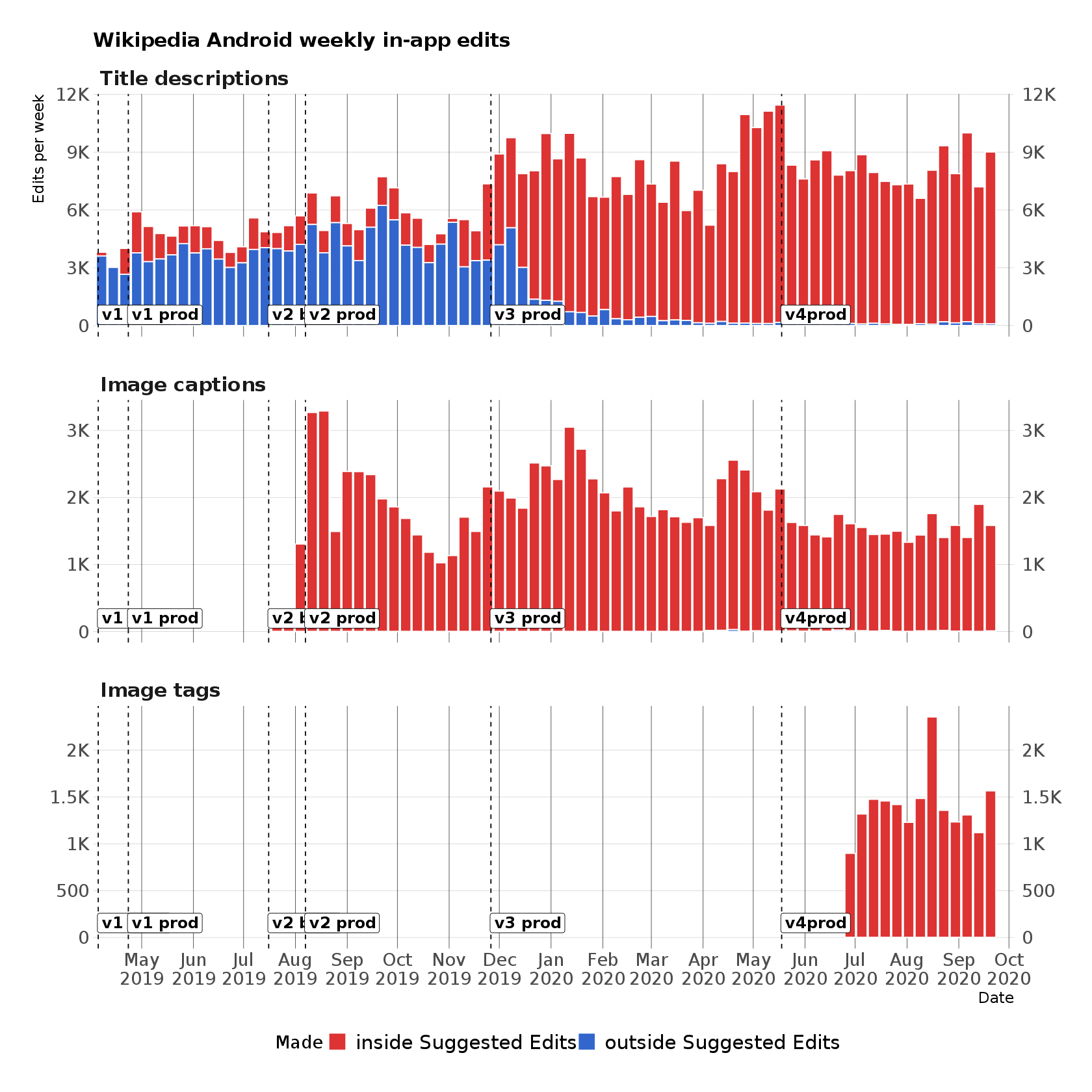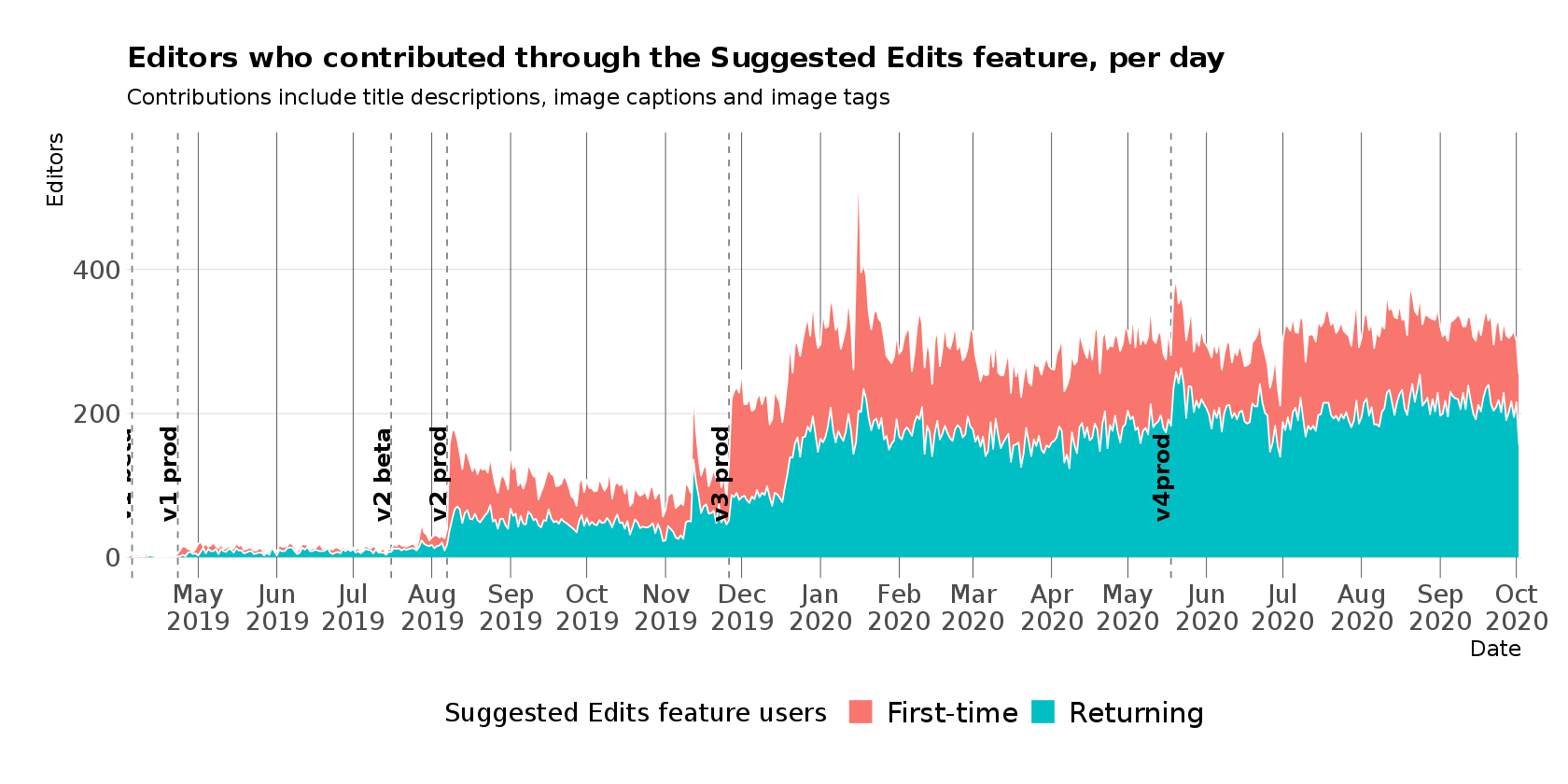In :
# https://stackoverflow.com/a/35018739/1091835
library(IRdisplay)

display_html(
'<script>
code_show=true;
function code_toggle() {
if (code_show){
$(\'div.input\').hide(); } else {$(\'div.input\').show();
}
code_show = !code_show
}
$( document ).ready(code_toggle); </script> <form action="javascript:code_toggle()"> <input type="submit" value="Click here to toggle on/off the raw code."> </form>' ) #To publish notebook #Run sugged_to_html.sh #jupyter nbconvert --ExecutePreprocessor.timeout=1800 --execute --to html SUGGED-v3.ipynb #mv SUGGED-v3.html suggested-edits-v2.html #cp suggested-edits-v2.html /srv/published/reports/wikipedia-android-app/ #published-sync  # Suggested Edits Reporting v3¶ ## Table of Contents¶ Note: Image tag data is included where possible but also shown in Superset here: https://superset.wikimedia.org/superset/dashboard/androidimagetags/ In : # Packages: library(glue) library(zeallot) library(magrittr) import::from(dplyr, group_by, keep_where = filter, ungroup, summarize, mutate, rename, select, arrange, n, left_join, distinct, count) import::from(tidyr, spread, gather) library(ggplot2) # library(ggrepel) import::from(wmf, theme_min, theme_facet) import::from(polloi, compress) library(repr) library(patchwork) #Getting dplyr error need to suppress options(dplyr.summarise.inform = FALSE)  In : # Helper functions: inf2na <- function(x) { y <- x y[is.infinite(x)] <- NA return(y) } nan2na <- function(x) { y <- x y[is.nan(x)] <- NA return(y) } na2zero <- function(x) { y <- x y[is.na(x)] <- 0 return(y) } suppress_messages_warnings <- function(x) { suppressMessages(suppressWarnings(x)) } to_html <- function(df, ...) { df %>% knitr::kable(format = "html", ...) %>% as.character() %>% IRdisplay::display_html() } options(warn = - 1)  In : #Added v3 and v4 - need data point added for v3 today <- Sys.Date() yesterday <- today - 1 feature_release_dates <- dplyr::tibble( date = as.Date(c("2019-04-05", "2019-04-23", "2019-07-16", "2019-08-07", "2019-11-26", "2020-05-18")), release = c("v1 beta", "v1 prod", "v2 beta", "v2 prod", "v3 prod", "v4prod") ) usage_date_range <- list( title_descriptions = c(feature_release_dates$date[feature_release_dates$release == "v1 prod"], yesterday), image_captions = c(feature_release_dates$date[feature_release_dates$release == "v2 prod"], yesterday), image_tags = c(feature_release_dates$date[feature_release_dates$release == "v4 prod"], yesterday) ) feature_release_dates <- keep_where(feature_release_dates, !is.na(date))  ## Edit stats¶ ### Edits and editors¶ In : editor_query <- " SELECT actor_name AS user_name, SUBSTR(rev_timestamp, 1, 8) AS date, SUM(IF(INSTR(comment_text, '#suggestededit') > 0 OR INSTR(comment_text, 'add-depict') > 0, 1, 0)) AS suggested_edits, COUNT(1) AS total_edits FROM revision LEFT JOIN revision_comment_temp rct ON revision.rev_id = rct.revcomment_rev LEFT JOIN comment ON rct.revcomment_comment_id = comment.comment_id LEFT JOIN change_tag ON revision.rev_id = change_tag.ct_rev_id LEFT JOIN revision_actor_temp rat ON revision.rev_id = rat.revactor_rev LEFT JOIN actor ON rat.revactor_actor = actor.actor_id${extra_join}
WHERE rev_timestamp >= '${rev_timestamp}' AND actor_user IS NOT NULL AND rat.revactor_actor > 0 -- remove anon edits (T188327 & T215466) AND ct_tag_id =${change_tag} -- android app edit
${extra_condition} GROUP BY user_name, date; " query_parameters <- list( wiki_db = c("title description" = "wikidatawiki", "image caption" = "commonswiki", "image tag" = "commonswiki"), rev_timestamp = c("20190401", "20190601", "20200518"), change_tag = c(14, 22, 22), extra_join = c("", "LEFT JOIN page ON revision.rev_page = page.page_id", "LEFT JOIN page ON revision.rev_page = page.page_id"), extra_condition = c("", "AND INSTR(comment_text, '* wbsetlabel-') > 0 AND page_namespace = 6", "AND INSTR(comment_text, 'add-depict') > 0 AND page_namespace = 6") ) fetch_editor_stats <- function(wiki_db, rev_timestamp, change_tag, extra_join, extra_condition) { editor_query <- glue(editor_query, .open = "${")
return(editor_stats)
}

editor_data <- purrr::pmap_dfr(query_parameters, fetch_editor_stats, .id = "edit_type") %>%
mutate(date = as.Date(date, "%Y%m%d")) %>%
keep_where(date < today)


Title descriptions, image captions and image tags made through the Suggested Edits feature have the comment "#suggestededit", which enables us to differentiate between those edits and regular edits (which can be made when reading an article).

The following is a breakdown of:

• how many title description, image caption & image tags edits have been made with the feature (and without it)
• how many editors have made Wikidata description edits, Commons image captions and Commons image tags through the Suggested Edits feature each day

This includes the Mobile Apps and Reading Infrastucture teams who have made contributions as part of the development and QA, prior to the public release of the beta and production versions of the app.

In :
made_with <- editor_data %>%
keep_where(date < today & date >= "2019-04-04") %>%
mutate(non_suggested_edits = total_edits - suggested_edits) %>%
group_by(edit_type, date) %>%
summarize(
outside Suggested Edits = sum(non_suggested_edits),
inside Suggested Edits = sum(suggested_edits)
) %>%
mutate(day = 1:n()) %>%
ungroup %>%
mutate(edit_type = factor(edit_type, c("title description", "image caption", "image tag"), c("Title descriptions", "Image captions", "Image tags")))
contributor_counts <- editor_data %>%
keep_where(date < today & date >= "2019-04-04") %>%
keep_where(suggested_edits > 0) %>%
arrange(user_name, date) %>%
group_by(user_name) %>%
mutate(returning = date > min(date)) %>%
ungroup %>%
dplyr::count(date, returning) %>%
mutate(returning = factor(returning, c(FALSE, TRUE), c("First-time", "Returning")))
edit_date_range <- list(
title_description = c(as.Date("2019-04-04"), yesterday),
image_caption = c(as.Date("2019-06-06"), yesterday),
image_tag = c(as.Date("2020-05-18"), yesterday))

In :
options(repr.plot.width = 14, repr.plot.height = 14)
gather(made, edits, -c(date, day, edit_type)) %>%
# aggregate by week:
mutate(date = lubridate::floor_date(date, "week")) %>%
summarize(edits = sum(edits), days = dplyr::n()) %>%
ungroup %>%
keep_where(days == 7) %>%
ggplot() +
geom_col(aes(x = date,  y = edits, fill = made), position = "stack", color = "white") +
geom_vline(aes(xintercept = date), data = feature_release_dates, linetype = "dashed", color = "black") +
geom_label(aes(x = date - 0.5, y = 50, label = release), data = feature_release_dates, size = 5,
vjust = "bottom", hjust = "left", fontface = "bold") +
scale_fill_manual(
values = c("inside Suggested Edits" = "#dd3333", "outside Suggested Edits" = "#3366cc")
) +
scale_x_date(date_breaks = "1 month", minor_breaks = NULL, date_labels = "%b\n%Y", expand = c(0, 2)) +
scale_y_continuous(minor_breaks = NULL, labels = compress,
sec.axis = sec_axis(~ ., labels = derive(), breaks = derive())) +
coord_cartesian(xlim = edit_date_range$title_description) + facet_wrap(~ edit_type, ncol = 1, scales = "free_y") + hrbrthemes::theme_ipsum("DejaVu Sans", base_size = 16, strip_text_face = "bold", strip_text_size = 18, caption_size = 12, axis_title_size = 14, subtitle_size = 14) + theme(legend.position = "bottom", panel.grid.minor.y = element_blank(), legend.text = element_text(size = 18), panel.grid.major.x = element_line(color = "gray10")) + labs(x = "Date", y = "Edits per week", fill = "Made", title = "Wikipedia Android weekly in-app edits")How to read these charts: on 8 April 2019, there were 3 total editors who collectively made 108 edits through the Editor Tasks (Suggested Edits) workflow. Of those 3 editors, 1 was somebody who made a contribution through Suggested Edits for the first time and 2 others were editors who have Suggested Edits-made contributions to their name already. In : options(repr.plot.width = 14, repr.plot.height = 7) ggplot(contributor_counts) + geom_vline(aes(xintercept = date), data = feature_release_dates, linetype = "dashed", color = "gray50") + geom_area(aes(x = date, y = n, fill = returning), position = "stack", color = "white") + geom_text(aes(x = date - 0.5, y = 50, label = release), data = feature_release_dates, size = 5, angle = 90, vjust = "bottom", hjust = "left", fontface = "bold") + scale_y_continuous(labels = compress, minor_breaks = NULL) + scale_x_date(date_breaks = "1 month", minor_breaks = NULL, date_labels = "%b\n%Y", expand = c(0, 1)) + coord_cartesian(xlim = edit_date_range$title_description) +
hrbrthemes::theme_ipsum("DejaVu Sans", base_size = 16, strip_text_face = "bold",
caption_size = 12, axis_title_size = 14, subtitle_size = 14) +
theme(legend.position = "bottom", legend.text = element_text(size = 18),
panel.grid.major.x = element_line(color = "gray10")) +
labs(x = "Date", y = "Editors", fill = "Suggested Edits feature users   ",
title = "Editors who contributed through the Suggested Edits feature, per day",
subtitles = "Contributions include title descriptions, image captions and image tags")### Title descriptions vs image captions vs image tags¶

Now that users can make two different types of edits (title descriptions and image captions), we can start to compare to the proportion of edits of one type versus the other. For our purposes, we do not differentiate between adding and translating.

In :
contributions_proportions <- made_with %>%
gather(made, edits, -c(date, day, edit_type)) %>%
select(date, contribution, edits) %>%
group_by(date) %>%
mutate(prop = edits / sum(edits)) %>%
ungroup %>%
group_by(contribution) %>%
arrange(date) %>%
mutate(day = 1:n()) %>%
ungroup

In :
options(repr.plot.width = 22, repr.plot.height = 10)
ggplot(contributions_proportions) +
geom_area(aes(x = date, fill = contribution, y = edits), position = "fill", color = "white") +
geom_hline(yintercept = seq(0, 1, 0.1), color = "white") +
scale_x_date(date_breaks = "1 month", minor_breaks = NULL, date_labels = "%b\n%Y", expand = c(0, 1)) +
scale_y_continuous(
minor_breaks = NULL, breaks = seq(0, 1, 0.1), labels = scales::percent_format(1),
sec.axis = sec_axis(~ 1 - ., labels = derive(), breaks = derive())
) +
scale_fill_brewer(palette = "Dark2") +
coord_cartesian(xlim = edit_date_range\$title_description) +
hrbrthemes::theme_ipsum("DejaVu Sans", base_size = 16, strip_text_face = "bold", plot_title_size = 24,
caption_size = 12, axis_title_size = 14, subtitle_size = 18) +
theme(legend.position = "bottom", panel.grid.minor.y = element_blank(), legend.text = element_text(size = 14)) +
labs(x = "Date", y = NULL, fill = "Edits",
title = "Breakdown of non-article in-app contributions by Android editors",
subtitles = "Contributions include title descriptions, image captions and image tags")